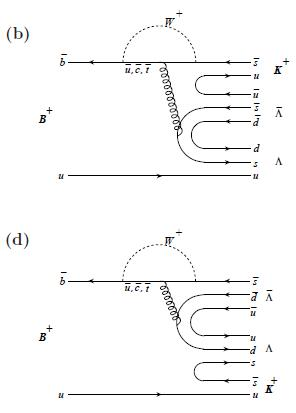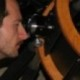## More ridiculously rare charmless decays from Belle November 30, 2008

Posted by dorigo in news, physics, science.
Tags: , ,

This morning I checked the Arxiv and found a paper just released by the Belle collaboration, an asymmetric electron-positron collider that runs at exactly the energy needed to produce B hadron pairs in large amounts and with small backgrounds. The paper deals with exactly the same topic of the CDF analysis I discussed in the former post yesterday, so I feel obliged to report its results here.

Belle does not observe bottom-strange mesons$B^\circ_s$, because the center-of-mass energy at which the collisions are produced (10.58 GeV, the mass of the$\Upsilon(4S)$ state) is not sufficient to create a pair of those mesons, which weigh about 80 MeV more than non-strange bottom mesons ($B^\circ, B^\pm$). Because of that, Belle must leave the study of the former to CDF and D0. However, the results it obtains on the latter are spectacularly accurate, thanks to the huge dataset (605 inverse femtobarns) it has accumulated.

One word about the size of this data sample: if compared with the few inverse femtobarns of proton-antiproton collisions produced by CDF, this seems a sample two hundred times larger of data. However, this is wrong. Remember, the master formula telling you how many events correspond, for a given physical process, to a given integrated luminosity is$N = \sigma L$

where$\sigma$ is the cross-section of the produced reaction, and L is the integrated luminosity the data correspond to. Now, while at the Tevatron collider one produces B hadrons with a cross section of a few microbarns, at Belle -and in general at electron-positron machines- the B production cross section is three orders of magnitude smaller. The result ? The number of B hadrons produced at the Tevatron is larger than that produced at Belle, despite the latter has 200 times more luminosity!

That said, to study non-strange bottom hadrons, electron-positron colliders are the machine to use. That is because the particles under study are produced in a much cleaner environment, with exactly the kinematics required to determine every detail of the production process precisely. At the Tevatron, instead, the large sample of B hadrons is produced more chaotically, with a large range of momenta, and collecting them is a challenge. Anyway, let us go back to Belle and its new observations.

Belle measures for the first time the decay of B mesons to very rare final states which do not involve a charm quark, like CDF. As I described yesterday, the typical product of a b-quark disintegration is a charm quark; much rarer is the direct$b \to u$ transition, and so the final state of B meson decay is very rarely devoid of charmed particles. Studying these rare decays provides sensitivity to possible deviations from the Standard Model, and is thus a very interesting thing to do.

The process studied is$B \to \Lambda \bar \Lambda h$, where$\Lambda$ is the strange baryon made up by a$uds$ triplet,$\bar \Lambda$ denotes its antiparticle state (made up by a$\bar u \bar d \bar s$ triplet), and h is any light meson such as a pion, a kaon, or some excited states of kaons. The interest of these decays, to me, lies in the rather complicated means by which baryons can materialize in a meson decay. In order to produce two three-(anti)quark states and a meson out of a meson decay, you must in fact “pick up” from the vacuum not just one, but as many as three quark-antiquark pairs!To see what I exactly mean, look at the two sample diagrams on the left, where you may think at time flowing from left to right. Each continuous line describes a quark propagation, while curly lines are gluons, and dashed ones are W bosons. You start with a B meson, a bound state of a b quark and a light antiquark state, and magically end up with two baryons and a meson. How can that happen ? Well, three quark-antiquark lines “pop” out of the vacuum, and bind orderly into the required final states.

The few of you really interested in the details of the experimental measurements of these rare reactions should read the paper directly, because I am not such an expert of B physics at electron-positron machines, and I will avoid administering inaccuracies here. I will only list below the results, which highlight the level of precision obtained by Belle:

•$B^\circ \to \Lambda \bar \Lambda K^\circ$:$BR= (4.76^{+0.84}_{-0.76} \pm 0.61) \times 10^{-6}$;
•$B^\circ \to \Lambda \bar \Lambda K^{\circ *}$:$BR=(2.46^{+0.87}_{-0.72} \pm 0.34) \times 10^{-6}$;
•$B^+ \to \Lambda \bar \Lambda K^+$:$BR=(3.38^{+0.41}_{-0.36}\pm 0.41) \times 10^{-6}$.

Other results for decay modes which Belle cannot yet claim to observe are also discussed in detail in the paper. One thing to note is that all these decays lie in the one-in-a-million range, much like those recently measured by CDF. This is the typical range of charmless decays of B mesons, which pay for the rarity of $b \to u$ transitions, as already discussed.

UPDATE: I should like to qualify the previous statement a bit, because I realize it is slightly misleading. Indeed, the rarity of$b \to u$ transitions is the reason why it is “penguin”-like diagrams such as those pictured in the graphs above the leading mechanisms by which B mesons decay to a charmless state! They do not involve, as a leading contribution,$b \to u$ transitions. Rather, they have a double weak transition$b \to c \to s$, as the W boson is first emitted and then reabsorbed by the quark line at the top.1. JJ - December 1, 2008

> Well, three quark-antiquark lines “pop” out of the vacuum

Surely only two from the vacuum, the third comes from the gluon decay…2. Dr B D O Adams - December 1, 2008

Those double lambda decays look like a great way to measure the
strong coupling constant. Would i’d be right in thinking that the rate
would go as alpha_strong^3, so the ratio of quark pickups decays to
ordinary di-meson decays should depend on alpha_strong^3 allowing an inference of alpha_strong to high accuracy.3. David Heffernan - December 4, 2008

> Belle does not observe bottom-strange mesons B^\circ_s, because the center-of-mass energy at which the collisions are produced (10.58 GeV, the mass of the \Upsilon(4S) state) is not sufficient to create a pair of those mesons,

Belle can – and has – observed B_s mesons by running the collider with a CM energy at the Y(5S) resonance. They have also done runs at Y(1S), Y(2S) and Y(3S), as well as energy scans in the bottomonium region. I believe the ratio of the energy (the boost) of the electron (HER) and positron (LER) beams is fixed, but it is possible to scale the CM energy. I think that theoretically the accelerator is certified up to about 10GeV x 5GeV ~ 14GeV in the CM.4. dorigo - December 4, 2008

That’s right, David, but it is not very meaningful to mention this in my post. Browse around here, and you’ll find many such “inaccuracies”. They are voluntary, and are meant to avoid distracting the reader from the important points… Here the important point is that B_s physics measurements are a Tevatron business.

Cheers,
T.

Sorry comments are closed for this entry